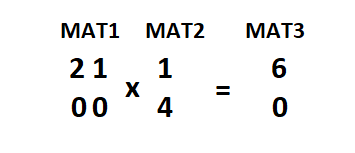# Matrix Multiplication

Posted: 14 Mar, 2021
Difficulty: Moderate

## PROBLEM STATEMENT

#### For example:

``````For the ‘MAT1’ and ‘MAT2’ given below, ‘MAT3’ is the matrix formed by multiplying ‘MAT1’ and ‘MAT2’.
````````````1. MAT3 = MAT1 * MAT2 + MAT1 * MAT2  ie. 2 * 1 + 1 * 4 = 6
2. MAT3 = MAT1 * MAT2 + MAT1 * MAT2 ie. 0 * 6 + 0 * 4 = 0
``````
##### Input Format:
``````The first line of input contains an integer ‘T’ denoting the number of test cases. Then each test case follows.

The first line of each test case contains four space-separated integers ‘N’, ‘M’, ‘M’, ‘P’ where ‘N’ and ‘M’ representing the number of rows and columns of ‘MAT1’ respectively and ‘M’ and ‘P’ representing the number of rows and columns of ‘MAT2’ respectively.

The next ‘N’ lines of each test case contain ‘M’ single space-separated integers denoting the values of ‘MAT1’. Then the next ‘M’ lines contain ‘P’ single space-separated integers denoting the values of ‘MAT2’
``````
##### Output Format :
``````For each test case, return the matrix ‘MAT3’ which will be formed by multiplying ‘MAT1’ and ‘MAT2’.
``````
##### Note:
``````You are not required to print the expected output; it has already been taken care of. Just implement the function.
``````
##### Constraints:
``````1 <= ‘T’ <= 100
1 <= ‘N’, ‘M’ and ‘P’ <= 100
-10^5 <= ‘MAT1[i][j]’ and ‘MAT2[i][j]’ <= 10^5

Time limit: 1 sec
``````Approach 1
• We can get the matrix ‘MAT3’ using 3 nested loops.
• To get the (‘i’, ‘j’) cell element of the matrix ‘MAT3’, we need to multiply each element of the ‘i’th row of ‘MAT1’ with the ‘j’’th column of the matrix ‘MAT2’ and finally add all the results.

Here is the complete algorithm:

• Make a 2D array/list ‘MAT3’ having ‘N’ rows and ‘P’ columns.
• Run a for loop from ‘i’ = 0 to ‘i’ < ‘N’ and for each ‘i’ do the following:
• Run a for loop from ‘j’ = 0 to ‘j’ < ‘P’ and for each ‘j’ do the following:
• Make a variable ‘sum’ = 0.
• Run a for loop from ‘k’ = 0 to ‘k’ < ‘M’ and for each ‘k’ do the following:
• sum’ = ‘sum’ + ‘MAT1[i][k]’ * ‘MAT2[k][j]’.
• Update ‘MAT3[i][j]’ = ‘sum’.
• Return ‘MAT3’.